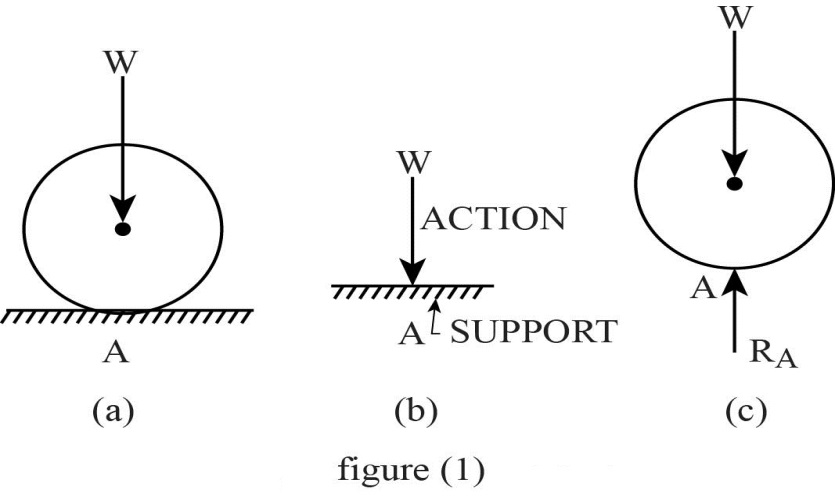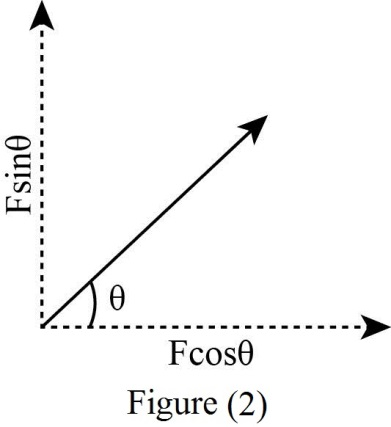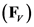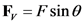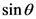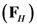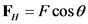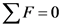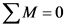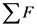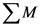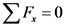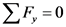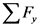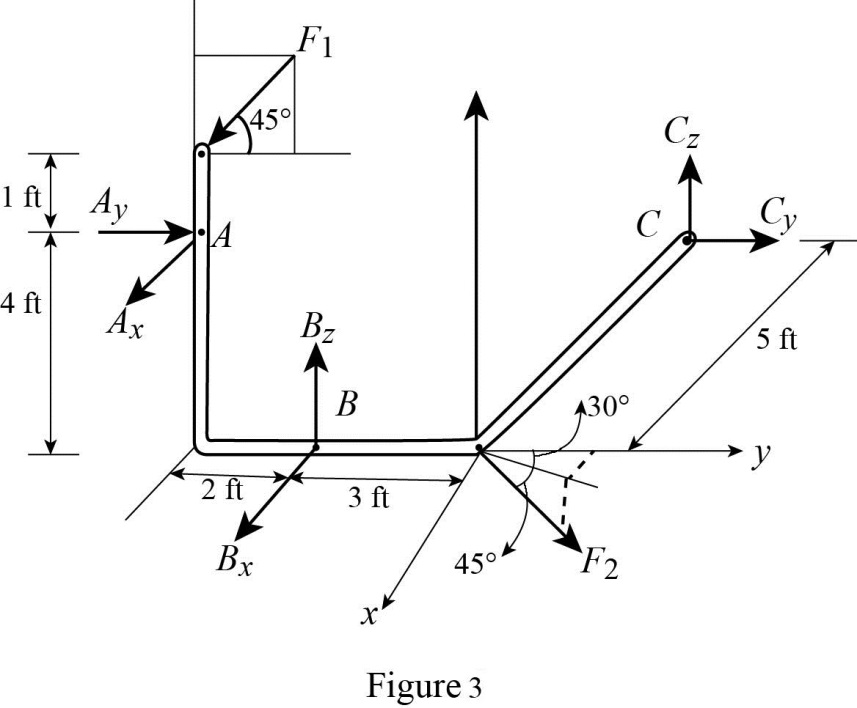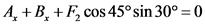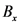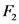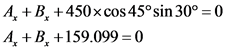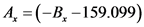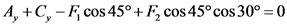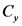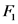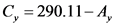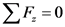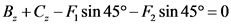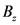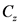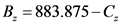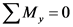# The bent rod is supported at a. b. and c by smooth journal bearings.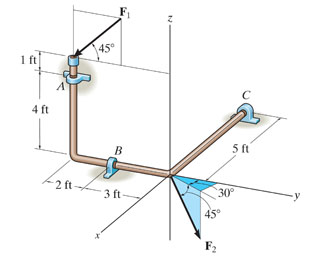The bent rod is supported at

A,
B, and
C by smooth journal bearings.
Compute the The bent rod is supported at
A ,
B , and
C by smooth journal bearings. Compute the
x,
y,
z components of reaction at the
bearing
A if the rod is subjected to forces

F1 = 800

lb
and
F2 =
450
lb.F1
lies in the
y?z plane. The bearings are in proper alignment and exert only
force reactions on the rod.
x ,
y ,
z components of reaction at the
bearing
A if the rod is subjected to forces
F1 F 1 = 800
lb l b and
F2 F 2 = 450
lb l b . F1 F 1 lies in the
y?z y ? z plane. The bearings are in proper alignment and exert only
force reactions on the rod. Compute the
x,
y,
z components of reaction at the
bearing
B. Compute the
x ,
y ,
z components of reaction at the
bearing
B . Compute the
x,
y,
z components of reaction at the
bearing
C.
Compute the
x ,
y ,
z components of reaction at the
bearing
C .

General guidance

Concepts and reason
Principle of equilibrium:
The principle of equilibrium states that, a stationary body will be in equilibrium when subjected to concurrent or parallel forces if the algebraic sum of all the external forces and moments of all the external forces is zero.
Free body diagram:
It is a graphical, symbolic illustration which is used to visualize the forces which are applied, moments and the reactions on a body for a particular condition.

Fundamentals

Action and Reaction:

On a horizontal surface, a ball is placed as shown in figure (1.a) which cannot move vertically downward but free to move along the horizontal surface. Therefore a force is exerted vertically downwards at the support by the ball as shown in figure (1.b) and this force is called as action.
At the point of contact of the ball with the surface, the support will exert a force vertically upwards on the ball as shown in figure (1.c) and this force is called as reaction.
Hence “any force on a support causes an equal and opposite force from the support so that action and reaction are two equal and opposite forces”.
Resolution of forces:
In resolving the forces as horizontal forces and vertical forces, when the angle of the force is made with the horizontal then the trigonometric angle of cosine is considered only in horizontal direction and the trigonometric angle of sine is considered only in vertical direction. The illustration is shown in Figure (2).

The vertical force is calculated as shown.

Here, force is F and angle of force with the normal is.
The horizontal force is calculated as shown.

Here, angle of force with the horizontal is.
Principle of equilibrium:
The equation for principal of equilibrium is written as,

Here, algebraic sum of the forces is , algebraic sum of the moments is , force law of equilibrium is , and moment law of equilibrium is .
In general, the forces are resolved into horizontal as well as vertical components.
The forces in the vertical components is written as,

The forces in the horizontal components is written as,

Here, algebraic sum of all horizontal components is , and algebraic sum of all vertical components is .
General sign convention for moment:
The moments which rotate the body in counter clockwise direction are taken as positive and the moments which rotate the body in clockwise direction are taken as negative.
General sign convention for force:
The forces in the rightward and upward direction are taken as positive while the forces in downward and leftward direction are taken as negative.

Step-by-step

Step 1 of 11

Draw the free body diagram of the system as shown in Figure (3).

Apply the force law of equilibrium along the x-direction.

Here, reaction at A along x-direction is , and reaction at B along x-direction is .
Substitute 450 lb for .

…… (1)
Apply the force law of equilibrium along the y-direction.

Here, reaction at A along y-direction is , and reaction at C along z-direction is .
Substitute 800 lb for and 450 lb for .

…… (2)
Apply the force law of equilibrium along the z-direction.

Here, reaction at B along z-direction is , and reaction at C along z-direction is .
Substitute 800 lb for and 450 lb for .

…… (3)

The free body diagram of the system is drawn and the force law of equilibrium is applied along x-axis, y-axis, and z-axis.

Do not make mistake while applying the force law of equilibrium along x-axis; consider only reactions along x-axis and forces inclined with x-axis.

Apply the moment law of equilibrium along x-axis, y-axis, and z-axis to form equations which are used to calculate the various reactions acting in the system.

Step 2 of 11

Apply the moment law of equilibrium along y-axis.

…… (4)
Apply the moment law of equilibrium along z-axis.

…… (5)
Apply the moment law of equilibrium along x-axis.

Substitute 800 lb for .

…… (6)

The equations are formed by applying the moment law of equilibrium along x-axis, y-axis, and z-axis which are used for calculating the various reactions in the system.

Do not make mistake in taking moment about the axis. The moments which rotate the body in counter clockwise direction are taken as positive and the moments which rotate the body in clockwise direction are taken as negative.

Calculate the reaction at B along x-direction using the equations obtained by applying the principle law of equilibrium.

Step 3 of 11

Find the reaction at B along x-direction from equation (5) as follows,

Substitute for from equation (1).

Substitute for from equation (2).

Substitute for from equation (6).

Substitute for from equation (3).

Substitute for from equation (4).

Substitute for from equation (1).

The reaction at B along x-direction is .

The reaction at B along x-direction is calculated using the equations obtained by applying the principle law of equilibrium.

Calculate the reaction at A along x-direction using the equation (1) obtained by applying the principle law of equilibrium.

Step 4 of 11

Find the reaction at A along x-direction from equation (1) as follows,

Substitute for .

The reaction at A along x-direction is .

The reaction at A along x-direction is calculated from the equation (1) by substituting the reaction at B along x-direction in the equation.

Do not make mistake in finding the value of . Use the correct relation as and not as .

Calculate the reaction at C along x-direction from the Figure (3).

Step 5 of 11

Find the reaction at C along x-direction.

The reaction at C along x-direction is 0 lb.

The reaction at C along x-direction is calculated from the Figure (3).

Calculate the reaction at A along x-direction from equation (4) obtained by applying the principle law of equilibrium.

Step 6 of 11

Find the reaction at C along z-direction from equation (4) as follows,

Substitute 1851.184 lb for .

The reaction at C along z-direction is .

The reaction at C along z-direction is calculated from equation (4) by substituting the reaction at A along x-direction in the equation.

Do not make mistake in finding the value of . Use the correct relation as and not as .

Calculate the reaction at B along z-direction from equation (3) obtained by applying the principle law of equilibrium.

Step 7 of 11

Find the reaction at B along z-direction from equation (3) as follows,

Substitute for .

The reaction at B along z-direction is .

The reaction at B along z-direction is calculated from equation (3) by substituting the value of the reaction at C along z-direction in the equation.

Do not make mistake in finding the value of . Use the correct relation as and not as .

Calculate the reaction at A along z-direction from the Figure (3).

Step 8 of 11

Find the reaction at A along z-direction.

The reaction at A along z-direction is 0 lb.

The reaction at A along z-direction is obtained from Figure (3).

Calculate the reaction at A along y-direction from equation (6) obtained by applying the principle law of equilibrium.

Step 9 of 11

Find the reaction at A along y-direction from equation (6) as follows,

Substitute for .

The reaction at A along y-direction is .

The reaction at A along y-direction is calculated from equation (6) by substituting the reaction at B along z-direction.

Do not make mistake in finding the value of . Use the correct relation as and not as .

Calculate the reaction at C along y-direction from equation (5) obtained by applying the principle law of equilibrium.

Step 10 of 11

Find the reaction at C along y-direction from equation (5) as follows,

Substitute for and for

The reaction at C along y-direction is .

The reaction at C along y-direction is calculated from equation (5) by substituting the reaction at A and B along x-direction.

Do not make mistake in finding the value of . Use the correct relation as and not as .

Calculate the reaction at B along y-direction from the Figure (3).

Step 11 of 11

Find the reaction at B along y-direction.

The reaction at B along y-direction is 0 lb.

The reaction at B along y-direction is obtained from Figure (3).

The reaction at B along x-direction is .

The reaction at A along x-direction is .

The reaction at C along x-direction is 0 lb.

The reaction at C along z-direction is .

The reaction at B along z-direction is .

The reaction at A along z-direction is 0 lb.

The reaction at A along y-direction is .

The reaction at C along y-direction is .

The reaction at B along y-direction is 0 lb.

The reaction at B along x-direction is .

The reaction at A along x-direction is .

The reaction at C along x-direction is 0 lb.

The reaction at C along z-direction is .

The reaction at B along z-direction is .

The reaction at A along z-direction is 0 lb.

The reaction at A along y-direction is .

The reaction at C along y-direction is .

The reaction at B along y-direction is 0 lb.

ACTION AL SUPPORT A TRA (a) (c) (b) figure (1)
Fsino Fcoso Figure (2)
F, = Fsino
F, = F cose
ΣF = 0
ΣΜ = 0
ΣF = 0
ΣΜ = 0
ΣF = 0
ΣΕ, = 0
1 ft| Ay 4 ft Ax 2 ff 3 ft / 450 F Figure 3
ΣF = 0
4+B+F, cos 45º sin 30º = 0
A + B +450x cos45°sin 30° = 0 4+B4 +159.099 = 0
ΣΕ, = 0
A, +C, – F, cos 45° + F, cos 45ºcos 30º = 0
4, +C, -800x cos 45º +450x cos 45ºcos 30º = 0 4,+C – 565.685+275.56=0 4, +C, – 290.11=0
B. = 883.875-C.
ΣΜ. = 0 A (2+ 3) + Β. (3)-C, (5) = 0
5656.85+3B. 45656
We were unable to transcribe this image